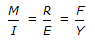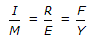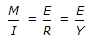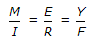# Civil Engineering - Theory of Structures - Discussion

### Discussion :: Theory of Structures - Section 3 (Q.No.5)

5.

If M, I, R, E, F, and Y are the bending moment, moment of inertia, radius of curvature, modulus of elasticity stress and the depth of the neutral axis at section, then

 [A].[B].[C].[D].Explanation:

No answer description available for this question.

 Vineet Shukla said: (Jul 24, 2015) m/i =f/y=e/r is correct.

 Dharmendra Kumar Sachan said: (Sep 4, 2016) IT is, F/Y = M/I = E/R.

 Pradyumna Kumar Nayak said: (Nov 4, 2016) Please correct the question. As in question, the option "C" is M/I = E/R = E/Y, But it should be M/I = E/R = F/Y. Actually, the last E/Y should be corrected to F/Y. The same question is there in AGOR book. There also the question is wrong. The answer option is "C".

 Chintu said: (Mar 8, 2017) It's M/I =f/y=E/R.

 Sunil Sharma said: (Apr 10, 2017) As per my knowledge, Instead E/Y there is F/F in answer.

 Pinki said: (Jun 23, 2017) I think it is M/I = E/R = F/Y.

 Sheela said: (Jul 23, 2017) Bending Moment Equation: M/I = F/Y = E/R.

 Akash said: (Oct 27, 2017) M/I=F/y=E/R.

 Muhammad Islam said: (Feb 24, 2018) It should be m/i=e/r=f/y.

 Vikash Parihar said: (Jun 29, 2018) It should be M/I=E/R=F/Y.

 Vikash Parihar said: (Jun 29, 2018) It should be M/I=E/R=F/Y.

 Vikash Parihar said: (Jun 29, 2018) It should be M/I=E/R=F/Y.

 Vikash Parihar said: (Jun 29, 2018) It should be M/I=E/R=F/Y.